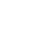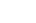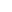## TS 10th class maths concept

Studying mathematics successfully meaning that, children take responsibility for their own learning and learn to apply the concepts to solve problems.

This note is designed by the ‘Basic In Maths’ team. These notes to do help students fall in love with mathematics and overcome fear.

## 1. REAL NUMBERS

• Rational number: The number, which is written in the form of p/q where p,q are integers q not equal to zero is called a rational number. It is denoted by Q.

• Irrational numbers:- the number, which is not rational is called an irrational number. It is denoted by Q’ or S.

• Euclid division lemma:- For any positive integers a and b, then q, r are integers exists uniquely satisfying the rules a = bq + r, 0 ≤ r < b.

• Prime number:- The number which has only two factors 1 and itself is called a prime number. (2, 3, 5, 7 …. Etc.)

• Composite number:- the number which has more than two factors is called a composite number. (4, 6, 8, 9, 10,… etc.)

• Co-prime numbers:- Two numbers  said to be co-prime numbers if they have no common factor except 1. [Ex: (1, 2), (3, 4), (4, 7)…etc.]

• To find HCF, LCM by using prime factorization method:  H. C.F= product of the smallest power of each common prime factors of given numbers. L.C.M = product of the greatest power of each prime factor of given numbers.

• In p/q, if prime factorisation of q is in form 2m 5n, then p/q is terminating decimal. Otherwise non-terminating repeating decimal.
• Decimal numbers with the finite no. of digits is called terminating Decimal numbers with the infinite no. of digits is called non-terminating decimal. In a decimal, a digit or a sequence of digits in the decimal part keeps repeating itself infinitely. Such decimals are called non-terminating repeating decimals.

• ‘p’ is a prime number and ‘a’ is a positive integer, if p divides a2, then p divides a.

• If ax = N then x =

(i) log (xy) = log(x) + log(y)  (ii) log (x/y) = log( x) – log( y) (iii) log (xm ) = m log (x)Loading...Taking too long?Reload document
|Open in new tab

Pair Of Linear Equations In two Variables Concept and Solutions: Click Here

### TS 10th class maths concept

Visit My Youtube Channel: Click on Below Logo